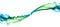# JavaScript Factory Functions with ES6+Smoke Art Cubes to Smoke — MattysFlicks — (CC BY 2.0)
`const user = {  userName: 'echo',  avatar: 'echo.png'};`
`console.log(user.userName); // "echo"`
`const key = 'avatar';console.log( user[key] ); // "echo.png"`
`const userName = 'echo';const avatar = 'echo.png';const user = {  userName,  avatar};console.log(user);// { "avatar": "echo.png",   "userName": "echo" }`
`const userName = 'echo';const avatar = 'echo.png';const user = {  userName,  avatar,  setUserName (userName) {    this.userName = userName;    return this;  }};console.log(user.setUserName('Foo').userName); // "Foo"`

# Literals for One, Factories for Many

`const createUser = ({ userName, avatar }) => ({  userName,  avatar,  setUserName (userName) {    this.userName = userName;    return this;  }});console.log(createUser({ userName: 'echo', avatar: 'echo.png' }));/*{  "avatar": "echo.png",  "userName": "echo",  "setUserName": [Function setUserName]}*/`

# Returning Objects

`const noop = () => { foo: 'bar' };console.log(noop()); // undefinedconst createFoo = () => ({ foo: 'bar' });console.log(createFoo()); // { foo: "bar" }`

# Destructuring

`const createUser = ({ userName, avatar }) => ({`
`const swap = ([first, second]) => [second, first];console.log( swap([1, 2]) ); // [2, 1]`
`const rotate = ([first, ...rest]) => [...rest, first];console.log( rotate([1, 2, 3]) ); // [2, 3, 1]`

# Computed Property Keys

`const key = 'avatar';console.log( user[key] ); // "echo.png"`
`const arrToObj = ([key, value]) => ({ [key]: value });console.log( arrToObj([ 'foo', 'bar' ]) ); // { "foo": "bar" }`
`{ [key]: value }`
`{ "foo": "bar" }`

# Default Parameters

`const createUser = ({  userName = 'Anonymous',  avatar = 'anon.png'} = {}) => ({  userName,  avatar});console.log(  // { userName: "echo", avatar: 'anon.png' }  createUser({ userName: 'echo' }),  // { userName: "Anonymous", avatar: 'anon.png' }  createUser());`
`} = {}) => ({`

# Factory Functions for Mixin Composition

`const withConstructor = constructor => o => ({  // create the delegate [[Prototype]]  __proto__: {    // add the constructor prop to the new [[Prototype]]    constructor  },  // mix all o's props into the new object  ...o});`
`import withConstructor from `./with-constructor';const pipe = (...fns) => x => fns.reduce((y, f) => f(y), x);// or `import pipe from 'lodash/fp/flow';`// Set up some functional mixinsconst withFlying = o => {  let isFlying = false;  return {    ...o,    fly () {      isFlying = true;      return this;    },    land () {      isFlying = false;      return this;    },    isFlying: () => isFlying  }};const withBattery = ({ capacity }) => o => {  let percentCharged = 100;  return {    ...o,    draw (percent) {      const remaining = percentCharged - percent;      percentCharged = remaining > 0 ? remaining : 0;      return this;    },    getCharge: () => percentCharged,    getCapacity: () => capacity  };};const createDrone = ({ capacity = '3000mAh' }) => pipe(  withFlying,  withBattery({ capacity }),  withConstructor(createDrone))({});const myDrone = createDrone({ capacity: '5500mAh' });console.log(`  can fly:  \${ myDrone.fly().isFlying() === true }  can land: \${ myDrone.land().isFlying() === false }  battery capacity: \${ myDrone.getCapacity() }  battery status: \${ myDrone.draw(50).getCharge() }%  battery drained: \${ myDrone.draw(75).getCharge() }% remaining`);console.log(`  constructor linked: \${ myDrone.constructor === createDrone }`);`

# Next Steps

Written by

Written by

## Eric Elliott

#### Make some magic. #JavaScript